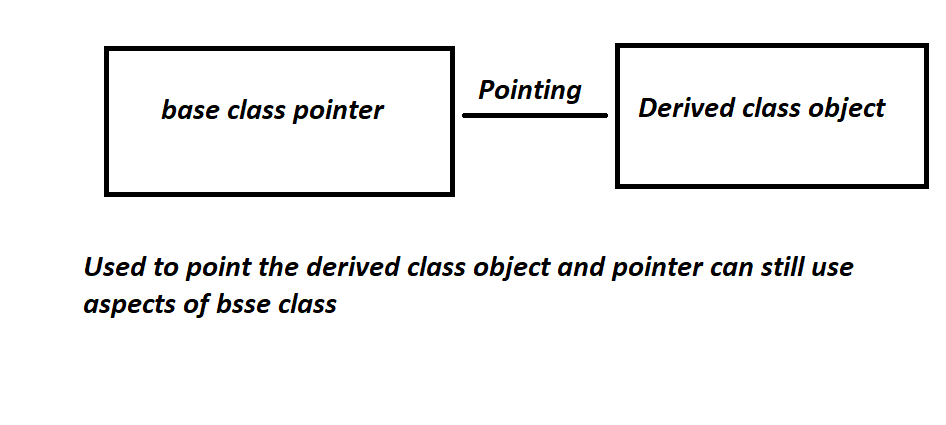# Base class pointer pointing to derived class object

• Difficulty Level : Easy
• Last Updated : 23 Aug, 2021

Pointer is a data type that stores the address of other data types.

The pointer of Base Class pointing different object of derived class:

Want to learn from the best curated videos and practice problems, check out the C++ Foundation Course for Basic to Advanced C++ and C++ STL Course for foundation plus STL.  To complete your preparation from learning a language to DS Algo and many more,  please refer Complete Interview Preparation Course.Approach:

• A derived class is a class which takes some properties from its base class.
• It is true that a pointer of one class can point to other class, but classes must be a base and derived class, then it is possible.
• To access the variable of the base class, base class pointer will be used.
• So, a pointer is type of base class, and it can access all, public function and variables of base class since pointer is of base class, this is known as binding pointer.
• In this pointer base class is owned by base class but points to derived class object.
• Same works with derived class pointer, values is changed.

Below is the C++ program to illustrate the implementation of the base class pointer pointing to the derived class:

## C++

 `// C++ program to illustrate the``// implementation of the base class``// pointer pointing to derived class``#include ``using` `namespace` `std;` `// Base Class``class` `BaseClass {``public``:``    ``int` `var_base;` `    ``// Function to display the base``    ``// class members``    ``void` `display()``    ``{``        ``cout << ``"Displaying Base class"``             ``<< ``" variable var_base: "` `<< var_base << endl;``    ``}``};` `// Class derived from the Base Class``class` `DerivedClass : ``public` `BaseClass {``public``:``    ``int` `var_derived;` `    ``// Function to display the base``    ``// and derived class members``    ``void` `display()``    ``{``        ``cout << ``"Displaying Base class"``             ``<< ``"variable var_base: "` `<< var_base << endl;``        ``cout << ``"Displaying Derived "``             ``<< ``" class variable var_derived: "``             ``<< var_derived << endl;``    ``}``};` `// Driver Code``int` `main()``{``    ``// Pointer to base class``    ``BaseClass* base_class_pointer;``    ``BaseClass obj_base;``    ``DerivedClass obj_derived;` `    ``// Pointing to derived class``    ``base_class_pointer = &obj_derived;` `    ``base_class_pointer->var_base = 34;` `    ``// Calling base class member function``    ``base_class_pointer->display();` `    ``base_class_pointer->var_base = 3400;``    ``base_class_pointer->display();` `    ``DerivedClass* derived_class_pointer;``    ``derived_class_pointer = &obj_derived;``    ``derived_class_pointer->var_base = 9448;``    ``derived_class_pointer->var_derived = 98;``    ``derived_class_pointer->display();` `    ``return` `0;``}`

Output:

```Displaying Base class variable var_base: 34
Displaying Base class variable var_base: 3400
Displaying Base classvariable var_base: 9448
Displaying Derived  class variable var_derived: 98```

Conclusion:

• A pointer to derived class is a pointer of base class pointing to derived class, but it will hold its aspect.
• This pointer of base class will be able to temper functions and variables of its own class and can still point to derived class object.

My Personal Notes arrow_drop_up